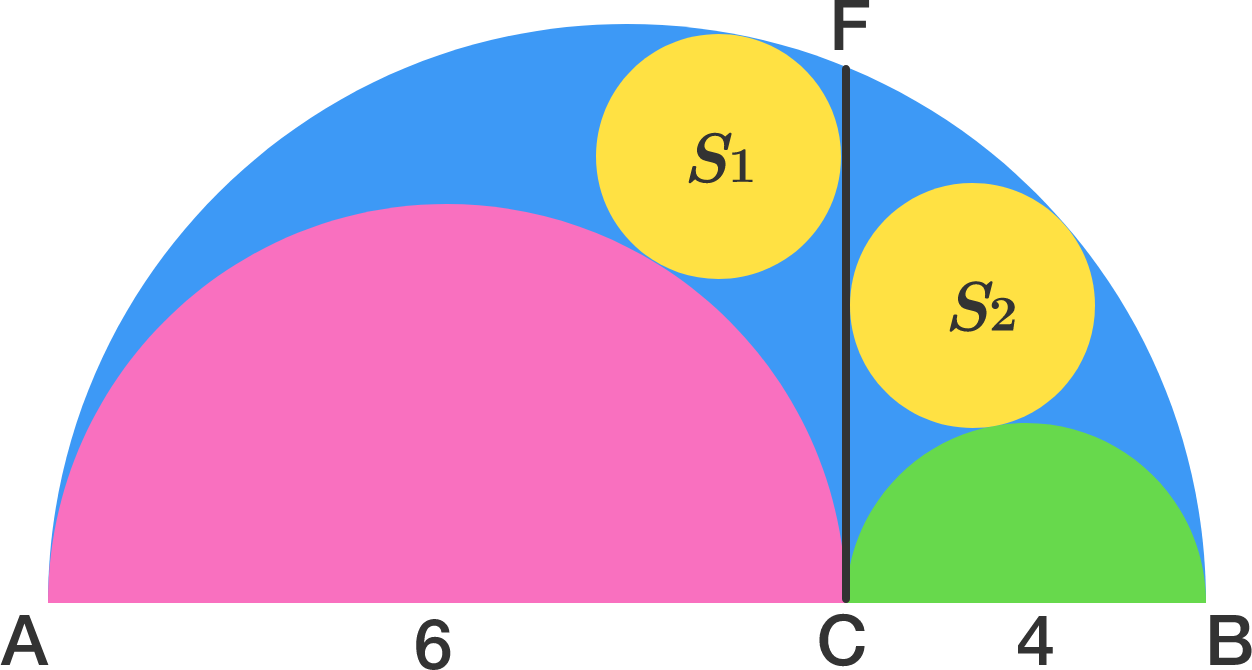# Archimedean Twins

Geometry Level 3In the figure there are three semicircles of diameters $AB$, $AC$, $BC$ and two circles $S_{1}$ and $S_{2}$ which are touching to the line $CF$ ( $CF$ is perpendicular to $AB$ ). Both circles have same radius and are called Archimedean Twins. Now if $AB = 10$, $AC = 6$ and $BC = 4$ then find the radius of circle $S_{1}$.

×

Problem Loading...

Note Loading...

Set Loading...